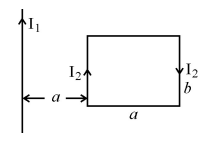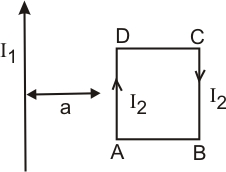# (a) Define the term ‘self inductance’ of a coil. Write its S.I. unit.(b)  A rectangular loop of sides a and b carrying currentis kept at a distance ‘a’ from an infinitely long straight wire carrying  currentas shown in the figure. Obtain an expression for the resultant force acting on the loop.Self-inductance (L) is the emf induced in the coil when the rate of change of current in the coil isampere/secondWhere= magnetic flux= Current flowing

Where= coefficient of self- inductance or self-inductance of the coil

when,Then,The S.I unit of self-inductance is Henry (H).

(b) We know the magnetic field due to the infinitely long current-carrying wire is given by :Where,= current carried by infinite wire= perpendicular distance of wire to that point.

Now, the force acting on a straight wire is given by,For side  ABin -ve x-direction.

Such that, for BC and AD forces are equal but opposite in direction so, they will cancel out each other.

Now, for side CDin +ve x- direction

Hence, the net force will be in -ve x-direction ,## Related Chapters

### Preparation Products

##### Knockout NEET May 2021 (One Month)

An exhaustive E-learning program for the complete preparation of NEET..

₹ 14000/- ₹ 6999/-
##### Foundation 2021 Class 10th Maths

Master Maths with "Foundation course for class 10th" -AI Enabled Personalized Coaching -200+ Video lectures -Chapter-wise tests.

₹ 350/- ₹ 112/-
##### Foundation 2021 Class 9th Maths

Master Maths with "Foundation course for class 9th" -AI Enabled Personalized Coaching -200+ Video lectures -Chapter-wise tests.

₹ 350/- ₹ 112/-
##### Knockout JEE Main April 2021 (One Month)

An exhaustive E-learning program for the complete preparation of JEE Main..

₹ 14000/- ₹ 6999/-